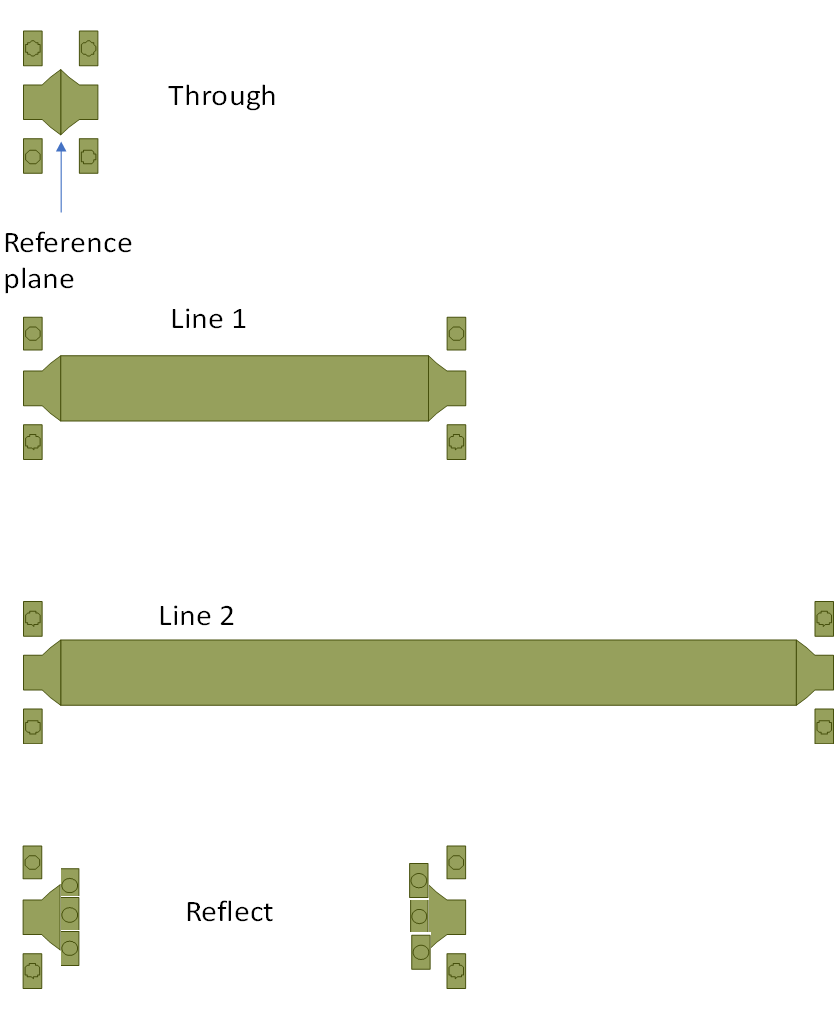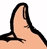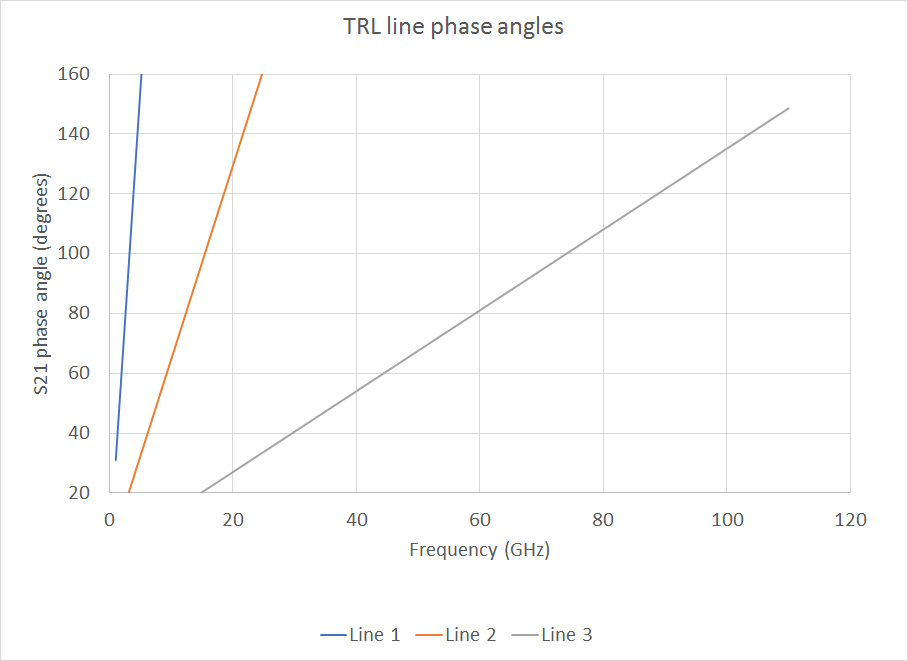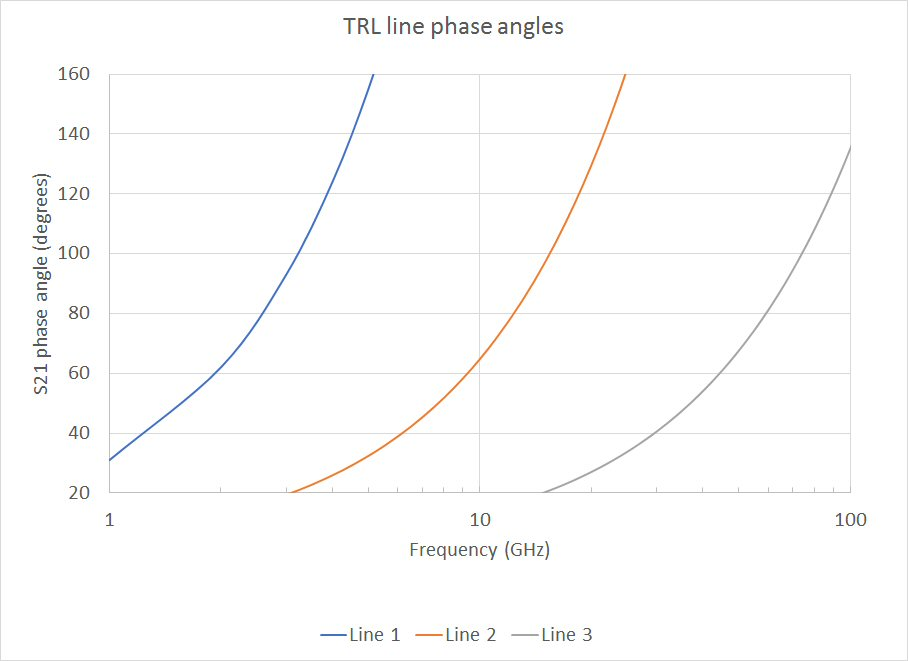# TRL calibration

Go to our download area and grab a free copy of our TRL calibration calculator spreadsheet, it will automatically calculate the best line lengths for any frequency range.

Through-reflect-line calibration is often used for de-embedding S-parameter data, especially on-wafer MMIC data. TRL works great, but it has a lower frequency limitation in that the "line" standard's length can be more than you'd want to fabricate.  We recommend using TRL for "normal" microwave frequencies (maybe down to a couple of GHz) and then using another scheme if you really need data down at 10 or 20 MHz.  For now, we are not going to get involved in the math for TRL calibration, and as an engineer, chances are you don't have to either.  But we will show you the best way to calculate line lengths for TRL cals, and we will follow up soon with a spreadsheet that does it all for you!

For this discussion, we are talking about de-embedding two-port data.  It is possible to de-embed three-port or higher-order data, but we will leave that for another day.

As the name implies, there are three standards that are measured: the "through" (sometimes called the "thru"), the reflect (often a short circuit or an open circuit) and the "line" (basically, the same as the thru, with some extra length inserted).

The image below is an artist's conception of a TRL calibration kit for a microstrip board that is RF probeable.  Any resemblance between this and real hardware is pure coincidence! On the top is the through, which is a back-to-back version of what you want to remove from the measurement ports. Each side of the through is referred to as a "launcher" or "launch", because it launches your test equipment signal into the device under test.  Note that it is really short... adding length increases errors. Certainly, the lengths of each side should be less than a quarter-wave at the uppermost frequency of calibration.

Below the three are two "lines" of different lengths. The ideal line is 1/4 wave in length, but if you measure over frequency, you don't want to make a quarterwave line for all data points.  It turns out that the line works well enough (engineering-speak for don't overthink this unless you are making a career out of accurately characterizing devices) if it is between 20 and 160 electrical degrees (a quarterwave is 90 degrees).  Depending on how much bandwidth you need to measure, you will probably need multiple lines cal standards. The longer ones are used at lower frequencies.

At the bottom is the "reflect", which is a short circuit in this case.  The reflection is ideally right at the reference planes of the launchers.  In the figure, two shorts (grounded with vias, the tiny circles) are configured to be the same distance between probes as line 1, to cut down on set-up. Two reflects are used, so you can calibrate both ports at once.  You could save some layout time by making one reflect standard, but the test guy/gal will wish that bad things happen to you as it forces them to have to turn the standard around during calibration.Here are some ways you can go wrong, in making calibration standards:

1. As we stated earlier, you can make the launchers really long (more than a quarterwave) and enjoy explaining away bad data at the high end of your band. We propose that you never exceed 1/8 wavelength for the launch.
2. Connectorized standards assume that the connectors behave exactly the same on every installation.  On PCBs, the assembler might not shove all the connectors up to the board (or maybe he/she will insist on a small gap, which could vary in length).
3. With connectorized standards, you can buy \$3 SMA connectors.  They will all be different, and in the best case you just get bad data.  In the worst case, they damage your VNA cables. Cough up for some precision connectors (line 2.92mm air dielectric) , that's why they were invented. And don't even think about making a cal kit with push-on connectors.
4. The reflect standard can bring misery... open circuits are notorious radiators, and short circuits have via inductance that brings significant reactance.  These problems get worse at high frequency, if you are trying to de-embed a 2.4 GHz amplifier you can't go wrong.  The best high-frequency standard you can make would be a "real" short circuit, where the launcher is terminated in a vertical wall of metal.  For microstrip circuits, you could make a metal shim the same thickness as the substrate and strap the microstrip line to it somehow.  Then add a wall of metal above the shim. Yes, we need to draw a picture of that one of these days.

If anyone has further advice on cal standards, send it in and we'll post it and maybe you will get a reward...

## The best way to calculate TRL "line" standard lengths

Before we get into this, one of the most annoying things about some engineers is that they don't pass along solutions to problems to other engineers, probably in an attempt to keep work flowing their way.  At many companies, there is the "TRL guy" that designs all the cal kits, because he/she keeps their mysterious method to themselves. Once you read the information below, you'll be in the know, too.Microwaves101 Rule of Thumb #126

The TRL line standard only works well when it is between 20 and 160 degrees electrical length.  This is just a rule of thumb, not a hard fact, but if your line standard is zero or 180 degrees in length, the math completely falls apart.  Considering the limit of 20 to 160 degrees, one line standard provides up to 156% bandwidth.  If you need more band, you'll need more than one standard, and your calibration routine will need to pick a crossover point for which lines are selected.

In this case, it's much more convenient to think of frequency coverage in terms of the ratio of upper to lower frequency: 20 to 160 degrees is 8:1 bandwidth, so a standard that is 20 degrees at 1 GHz will work to 8 GHz. Let's see how much bandwidth you can get by multiple standards:

Two standards can cover 64:1 bandwidth

Three standards can cover 512:1 bandwidth

Four standards can cover 4096:1 bandwidth

Can you figure out how much band can be covered by five standards?  Here's a hint: it's more than you will ever need, so give it up at four standards. Also, the low frequency standard can get extremely long for MHz frequencies.  How are you going to make a one-meter standards on a 100mm GaAs wafer?

Put another way, suppose you wanted to measure to 110 GHz, what would be the lowest frequency you could calibrate?

One standard can cover down to 13.75 GHz

Two standards can cover down to 1.72 GHz

Three standards can cover down to 215 MHz

Four standards can cover down to 27 MHz

We will use an example to describe the technique.  You have been assigned to design 1-110 GHz standards on GaAs (Keff ~8.25). First, you will notice that the band is 110:1, so three standards are needed.  The easiest thing to do is to come up with a standard that is 20 degrees at 1 GHz and a standard that is 160 degrees at 110 GHz, and somehow come up with a standard in the middle.  Maybe you just geometrically average (not arithmetically average) Line 1 and Line 3 to get Line 2.  This takes a few minutes and you can be done.  But suppose you want the very best calibration?  You will want to design standards and crossover points that ensure you are as far from 0 and 180 degrees as mathematically possible across the entire band.

First, you need to calculate the best crossover points.  This is done by geometric (as opposed to arithmetic) segmentation of the band. For two standards, the crossover is found by:

FT=FL*10^(log10(FH/FL)*(1/2))

Which simplifies to

FT=(FH/FL)^(1/2)

For three standards, crossovers FT1 and FT2 are:

FT1=FL*10^(log10(FH/FL)*(1/3))

FT2=FL*10^(log10((FH/FL)*(2/3))

(Apologies to anyone that tried the original math we posted, it has been fixed for May 2018.  You'll never need to set up the equations yourself, it is built into our TRL spreadsheet you can download...)

Notice the pattern?  You can figure out how to do four or five standards yourself.  Where did we get these formulas?  We figured them out in our spare time, they don't appear in any reference we could find.

For the 1-110 GHz example, crossover 1 is 4.79 GHz and crossover 2 is 22.96 GHz

Now, calculate the center frequency of each sub-band.  This is the arithmetic average of the adjacent crossover points.

For band 1, FC=1+(4.79-1)/2=2.895 GHz

For band 2, FC=4.79+(22.96-4.79)/2=13.87 GHz

For band 3, FC=22.96+(110-22.96)/2=66.48 GHz

Now, calculate quarterwave line lengths for each center frequency, these are the what you will need to fabricate.  Don't forget to take the effective dielectric constant (8.25) into account.

For band 1 (line 1), L=300/2.89/SQRT(8.25)/4=9.02mm

For band 2 (line 2), L=300/13.87/SQRT(8.25)/4=1.88mm

For band 3 (line 3), L=300/66.48/SQRT(8.25)/4=0.39mm

Now, let's plot phase angles of the three cal standards.  At 1 GHz, line 1 is 30 degrees, and at 110 GHz, line 3 is ~150 degrees.  Both of these points are 10 degrees inside the 20-160 degree rule of thumb.  But when you plot on a linear axis, it is hard to see the crossover points...Let's re-plot that on log scale. If you put a vertical line at the crossover points, you'd see that the phases are all between 30 and 150 degrees.Now you know the how to design a TRL cal kit, picking the best crossover frequencies and line lengths!

## An Alternate Opinion

We recently got a note from Shankar, who disagrees with us on one point.

" I guess I found an error in this article.  It stresses that the THRU length should always be smaller than 1/4th of the wavelength. I believe this is incorrect. In fact,  the opposite is true. THRU length should be approximately 40% more than the wavelength to avoid multiple modes getting excited."

And he included a couple of references with links (note: these links point to the IEEExplore site, which may or may not let you see more than the abstract depending on your subscription status):

Orii et al., "On the length of THRU standard for TRL de-embedding on Si substrate above 110 GHz," 2013 IEEE International Conference on Microelectronic Test Structures (ICMTS), Osaka, Japan, 2013, pp. 81-86, doi: 10.1109/ICMTS.2013.6528150.

D. F. Williams, R. B. Marks, and A. Davidson, "Comparison of on-wafer calibrations," 38th ARFTG Conf., pp. 68-81, Dec. 1991.

D. F. Williams and R. B. Marks, "Calibrating on-wafer probes to the probe tips," 40th ARFTG Conf., pp. 136-143, Dec. 1992.

So, what do you think?

Author : Unknown Editor